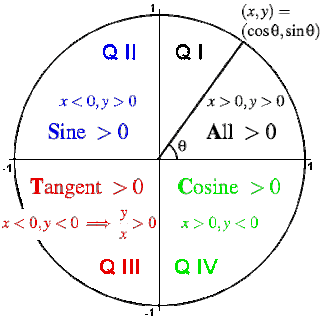Friday, September 25, 2009

Signs of sine, cosine and tangent, by Quadrant

The definition of the trigonometric functions cosine and sine in terms the coordinates of points lying on the unit circle tell us the signs of the trigonometric functions in each of the four quadrants, based on the signs of the x and y coordinates in each quadrant.For an angle in the first quadrant the point P has positive x and y coordinates. Therefore: In Quadrant I, cos(θ) > 0, sin(θ) > 0 and tan(θ) > 0 (All positive).

For an angle in the second quadrant the point P has negative x coordinate and positive y coordinate. Therefore: In Quadrant II, cos(θ) < 0, sin(θ) > 0 and tan(θ) < 0 (Sine positive).

For an angle in the third quadrant the point P has negative x and y coordinates. Therefore: In Quadrant III, cos(θ) < 0, sin(θ) < 0 and tan(θ) > 0 (Tangent positive).

For an angle in the fourth quadrant the point P has positive x coordinate and negative y coordinate. Therefore: In Quadrant IV, cos(θ) > 0, sin(θ) < 0 and tan(θ) < 0 (Cosine positive).

The quadrants in which cosine, sine and tangent are positive are often remembered using a favorite mnemonic.
One example: All Students Take Calculus.

1.2.